Please start any new threads on our new site at https://forums.sqlteam.com. We've got lots of great SQL Server experts to answer whatever question you can come up with.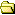All Forums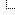Site Related Forums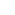The Yak Corral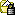Password Generation Challenge

Author  Topic

SamC
White Water Yakist

3467 Posts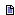Posted - 2005-12-15 : 15:58:34 I've seen JavaScripts and C do this... how about SQL?Generate a unique 8 character password using SQL containing upper, lower, numeric and special characters using the fewest possible statements.edit: Maybe I meant "random" not "unique"...

robvolk
Most Valuable Yak

15732 PostsPosted - 2005-12-15 : 16:00:13 SELECT CAST(CAST(newid() as binary(16)) as varchar(8))What do I win?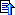blindman
Master Smack Fu Yak Hacker

2365 PostsPosted - 2005-12-15 : 16:00:39 Completely random?How about bonus points if the resulting passwords are extremely difficult to type?blindman
Master Smack Fu Yak Hacker

2365 PostsPosted - 2005-12-15 : 16:02:37 quote:Originally posted by robvolkSELECT CAST(CAST(newid() as binary(16)) as varchar(8))What do I win?Well, you definitely get the bonus points...TG
Master Smack Fu Yak Hacker

6065 PostsPosted - 2005-12-15 : 16:03:40 Sam if you want a password generator just say so. You need to call it a "challenge"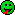Here's one I wrote for myself at some point. I wasn't going for least statements though but it does the upper, lower, numeric, special masking:`/*Returns a password with a similar mask as the passed in templatemask consists of length, upper case, lower case, numbers, and special charactersso these have the same mask:zFp8=B

blindman
Master Smack Fu Yak Hacker

2365 PostsPosted - 2005-12-15 : 16:18:55 Here is one I just cooked up:`select char(94 * RAND() + 33) + char(94 * RAND() + 33) + char(94 * RAND() + 33) + char(94 * RAND() + 33) + char(94 * RAND() + 33) + char(94 * RAND() + 33) + char(94 * RAND() + 33) + char(94 * RAND() + 33)`Michael Valentine Jones
Yak DBA Kernel (pronounced Colonel)

7020 PostsPosted - 2005-12-15 : 16:42:49 Well this isn't a short one, but it generates a password from a list of characters you can change with a random password length between the min and max you define. I removed the characters like zero and O, one and l and others that are easy to confuse. You can add or remove extra copies of the letter, numbers, and special characters to weight it towards one or the other. It also generates a stored procedure call to change the password if you need it for a SQL Server password. Kind of geeked out really.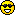`/* Script: Generate_Password.sqlThis script generates a random password made up ofupper and lowercase letters, numbers, and special characters.It also generates a SQL Server password changestored procedure call.To use this script in Query Analyzer1. Set the minimum password length, @PASSWORD_MIN_LENGTH2. Set the maximum password length, @PASSWORD_MAX_LENGTH3. Set the login name, @LOGIN4. Execute the script */set nocount ondeclare @PASSWORD_MIN_LENGTH intdeclare @PASSWORD_MAX_LENGTH intdeclare @LOGIN SYSNAMEselect @PASSWORD_MIN_LENGTH = 12select @PASSWORD_MAX_LENGTH = 20select @LOGIN = 'mylogin'declare @PASSWORD_DATA table (PASSWORD_CHAR VARCHAR(1) )declare @NUM table ( DIGIT int not null primary key clustered)insert into @NUM (DIGIT)select DIGIT = 0 union all select DIGIT = 1 union allselect DIGIT = 2 union all select DIGIT = 3 union allselect DIGIT = 4 union all select DIGIT = 5 union allselect DIGIT = 6 union all select DIGIT = 7 union allselect DIGIT = 8 union all select DIGIT = 9order by 1insert into @PASSWORD_DATA (PASSWORD_CHAR)select PASSWORD_CHAR = substring(b.CHARACTERS,a.RAND_INT%b.MOD,1)from(select aa.NUMBER, RAND_INT = abs(convert(int,convert(varbinary(100),newid())))from ( select NUMBER = a.DIGIT+(b.DIGIT*10) from @NUM a cross join @NUM b ) aa) across join(select MOD = len(bb.CHARACTERS)-1, bb.CHARACTERSfrom ( select CHARACTERS = 'ABCDEFGHJKLMNPQURSUVWXYZ'+ 'abcdefghjkmnpqursuvwxyz'+ 'ABCDEFGHJKLMNPQURSUVWXYZ'+ 'abcdefghjkmnpqursuvwxyz'+ 'ABCDEFGHJKLMNPQURSUVWXYZ'+ 'abcdefghjkmnpqursuvwxyz'+ 'ABCDEFGHJKLMNPQURSUVWXYZ'+ 'abcdefghjkmnpqursuvwxyz'+ '23456789'+ '23456789'+ '23456789'+ '23456789'+ '23456789'+ '23456789'+ '23456789'+ '23456789'+ '23456789'+ '23456789'+ '@#\$^*+=<>?'+ '@#\$^*+=<>?'+ '@#\$^*+=<>?'+ '@#\$^*+=<>?' ) bb) border by newid()--select * from @PASSWORD_DATAdeclare @password varchar(100)select @password = ''select @password = @password+PASSWORD_CHARfrom @PASSWORD_DATAselect @password = -- Random length from MIN to MAX characters substring(@password,1, @PASSWORD_MIN_LENGTH + (abs(convert(int,convert(varbinary(100),newid()))))%(@PASSWORD_MAX_LENGTH -@PASSWORD_MIN_LENGTH+1)) print 'PASSWORD = '+@password+'exec master.dbo.sp_password @old = NULL , @new = '''+@password+''', @loginame = '''+isnull(@LOGIN,'NULL')+''''`Script Output`PASSWORD = 9dHCR8hfDpU85dYbrEA*exec master.dbo.sp_password @old = NULL , @new = '9dHCR8hfDpU85dYbrEA*', @loginame = 'mylogin'`CODO ERGO SUMSamC
White Water Yakist

3467 PostsPosted - 2005-12-15 : 16:50:16 quote:Originally posted by robvolkSELECT CAST(CAST(newid() as binary(16)) as varchar(8))What do I win?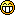SamC
White Water Yakist

3467 PostsPosted - 2005-12-15 : 16:53:13 quote:Originally posted by blindmanHere is one I just cooked up:`select char(94 * RAND() + 33) + char(94 * RAND() + 33) + char(94 * RAND() + 33) + char(94 * RAND() + 33) + char(94 * RAND() + 33) + char(94 * RAND() + 33) + char(94 * RAND() + 33) + char(94 * RAND() + 33)`Very simple! Reasonable results, though it won't guarrantee a mix of all character types.Michael Valentine Jones
Yak DBA Kernel (pronounced Colonel)

7020 PostsPosted - 2005-12-15 : 16:54:17 quote:Originally posted by robvolkSELECT CAST(CAST(newid() as binary(16)) as varchar(8))What do I win?You get your password set to this:Í#òÍuDThat should be reward enough.CODO ERGO SUMSamC
White Water Yakist

3467 PostsPosted - 2005-12-15 : 16:57:19 Michaels does the job, but it's long. Some great ideas though.SamC
White Water Yakist

3467 PostsPosted - 2005-12-15 : 16:59:04 TG - Yours seems to meet the requirements... fewer lines of code too...I gotta take some time to study both yours and Michael's with a couplas in hand.Michael Valentine Jones
Yak DBA Kernel (pronounced Colonel)

7020 PostsPosted - 2005-12-15 : 17:17:03 Just for fun, I "borrowed" you idea, and "enhanced" it with code from mine, and added the REPLICATE and WHILE to make it shorter. You can still generate a password of random length whithin the range of MIN to MAX, and define a weighted list of characters to select from.`declare @chars varchar (8000)declare @pass varchar (100)declare @cnt intdeclare @len intdeclare @min intdeclare @max intdeclare @pw_len intselect @min = 10, @max = 15select @pw_len = @min + convert(int,rand()*(@max-@min+1))select @chars =replicate('ABCDEFGHJKLMNPQURSUVWXYZ',8)+replicate('abcdefghjkmnpqursuvwxyz',8)+replicate('23456789',9)+replicate('@#\$^*+=<>?',4)select @len = len(@chars)-1select @cnt = 0, @pass = ''while @cnt < @pw_len begin set @cnt = @cnt + 1 select @pass = @pass + substring(@chars,convert(int,rand()*@len),1) end select pass = @pass`Output:`pass ------------- r8e4FfvMZDrX<(1 row(s) affected)`quote:Originally posted by blindmanHere is one I just cooked up:`select char(94 * RAND() + 33) + char(94 * RAND() + 33) + char(94 * RAND() + 33) + char(94 * RAND() + 33) + char(94 * RAND() + 33) + char(94 * RAND() + 33) + char(94 * RAND() + 33) + char(94 * RAND() + 33)`CODO ERGO SUMMichael Valentine Jones
Yak DBA Kernel (pronounced Colonel)

7020 PostsPosted - 2005-12-15 : 20:44:08 Getting back to the original password generation challenge, this code generates the 8 character random password containing upper, lower, numeric and special characters with only four statements.`declare @ch varchar (8000), @ps varchar (10)select @ps = '', @ch =replicate('ABCDEFGHJKLMNPQURSUVWXYZ',8)+replicate('23456789',9)+replicate('abcdefghjkmnpqursuvwxyz',8)+replicate('@#\$^*+=<>?',6)while len(@ps)<8 begin set @ps=@ps+substring(@ch,convert(int,rand()*len(@ch)-1),1) endselect [Password] = @ps`quote:Originally posted by SamCI've seen JavaScripts and C do this... how about SQL?Generate a unique 8 character password using SQL containing upper, lower, numeric and special characters using the fewest possible statements.edit: Maybe I meant "random" not "unique"...CODO ERGO SUMSamC
White Water Yakist

3467 PostsPosted - 2005-12-15 : 20:59:54 quote:Originally posted by Michael Valentine Jones`declare @ch varchar (8000), @ps varchar (10)select @ps = '', @ch =replicate('ABCDEFGHJKLMNPQURSUVWXYZ',8)+replicate('23456789',9)+replicate('abcdefghjkmnpqursuvwxyz',8)+replicate('@#\$^*+=<>?',6)while len(@ps)<8 begin set @ps=@ps+substring(@ch,convert(int,rand()*len(@ch)-1),1) endselect [Password] = @ps`Is this a great forum or what?Michael Valentine Jones
Yak DBA Kernel (pronounced Colonel)

7020 PostsPosted - 2005-12-15 : 22:23:52 I have to stop playing with this. This does it in one statement.`select [Password] = substring(ch,convert(int,rand()*len(ch)-1),1)+ substring(ch,convert(int,rand()*len(ch)-1),1)+ substring(ch,convert(int,rand()*len(ch)-1),1)+ substring(ch,convert(int,rand()*len(ch)-1),1)+ substring(ch,convert(int,rand()*len(ch)-1),1)+ substring(ch,convert(int,rand()*len(ch)-1),1)+ substring(ch,convert(int,rand()*len(ch)-1),1)+ substring(ch,convert(int,rand()*len(ch)-1),1)from (select ch = replicate('ABCDEFGHJKLMNPQURSUVWXYZ',8)+ replicate('abcdefghjkmnpqursuvwxyz',8)+ replicate('23456789',9)+replicate('@#\$^*+=<>?',7) ) a`Output:`Password -------- j+vPGNB4(1 row(s) affected)`CODO ERGO SUMelwoos
Master Smack Fu Yak Hacker

2052 PostsPosted - 2005-12-16 : 03:16:43 Where's Igor when you need him, I bet he has an answer to thissteve-----------Facts are meaningless. You could use facts to prove anything that's even remotely true!elwoos
Master Smack Fu Yak Hacker

2052 PostsPosted - 2005-12-16 : 03:21:45 quote:select [Password] = substring(ch,convert(int,rand()*len(ch)-1),1)+ substring(ch,convert(int,rand()*len(ch)-1),1)+ substring(ch,convert(int,rand()*len(ch)-1),1)+ substring(ch,convert(int,rand()*len(ch)-1),1)+ substring(ch,convert(int,rand()*len(ch)-1),1)+ substring(ch,convert(int,rand()*len(ch)-1),1)+ substring(ch,convert(int,rand()*len(ch)-1),1)+ substring(ch,convert(int,rand()*len(ch)-1),1)from (select ch = replicate('ABCDEFGHJKLMNPQURSUVWXYZ',8)+ replicate('abcdefghjkmnpqursuvwxyz',8)+ replicate('23456789',9)+replicate('@#\$^*+=<>?',7) ) aMore seriously, I think there is a minor bug in this as when I was just playing around with it one of the results I got was a NULLI just need to work out how now! It's when rand() returns 0 (or close enough that it is interpreted as 0) so something like substring(ch,convert(int,(rand()+1)*len(ch)-1),1)+works I thinksteve-----------Facts are meaningless. You could use facts to prove anything that's even remotely true!khtan
In (Som, Ni, Yak)

17689 PostsPosted - 2005-12-16 : 04:38:19 quote:More seriously, I think there is a minor bug in this as when I was just playing around with it one of the results I got was a NULLquote:It's when rand() returns 0 (or close enough that it is interpreted as 0) but substring(ch, -1, 1) will not return NULL but empty string. So the effect will be the password generated is 7 chars intead of 8 (if only one of the rand() returns 0).I tried running the codes 1 mil times and did not get NULL password at all however, i do get several 7 chars password generated.-----------------[KH]Learn something new everydayMichael Valentine Jones
Yak DBA Kernel (pronounced Colonel)

7020 PostsPosted - 2005-12-16 : 07:45:11 Here it is using NEWID() to generate the random number. Don't feel like figuring out that RAND() problem.I tested this code with 10 million passwords, and got 8 character passwords each time. If there is a bug in this one, it's not showing up very often.`select[Password] =substring(ch,(abs(convert(int,convert(varbinary(20),newid())))%len(ch))+1,1)+substring(ch,(abs(convert(int,convert(varbinary(20),newid())))%len(ch))+1,1)+substring(ch,(abs(convert(int,convert(varbinary(20),newid())))%len(ch))+1,1)+substring(ch,(abs(convert(int,convert(varbinary(20),newid())))%len(ch))+1,1)+substring(ch,(abs(convert(int,convert(varbinary(20),newid())))%len(ch))+1,1)+substring(ch,(abs(convert(int,convert(varbinary(20),newid())))%len(ch))+1,1)+substring(ch,(abs(convert(int,convert(varbinary(20),newid())))%len(ch))+1,1)+substring(ch,(abs(convert(int,convert(varbinary(20),newid())))%len(ch))+1,1)from(select ch =replicate('ABCDEFGHJKLMNPQURSUVWXYZ',8)+replicate('abcdefghjkmnpqursuvwxyz',8)+replicate('23456789',9)+replicate('@#\$^*+=<>?',7) ) a`CODO ERGO SUMSamC
White Water Yakist

3467 PostsPosted - 2005-12-16 : 07:56:41 quote:Originally posted by Michael Valentine Jones`select[Password] =substring(ch,(abs(convert(int,convert(varbinary(20),newid())))%len(ch))+1,1)+substring(ch,(abs(convert(int,convert(varbinary(20),newid())))%len(ch))+1,1)+substring(ch,(abs(convert(int,convert(varbinary(20),newid())))%len(ch))+1,1)+substring(ch,(abs(convert(int,convert(varbinary(20),newid())))%len(ch))+1,1)+substring(ch,(abs(convert(int,convert(varbinary(20),newid())))%len(ch))+1,1)+substring(ch,(abs(convert(int,convert(varbinary(20),newid())))%len(ch))+1,1)+substring(ch,(abs(convert(int,convert(varbinary(20),newid())))%len(ch))+1,1)+substring(ch,(abs(convert(int,convert(varbinary(20),newid())))%len(ch))+1,1)from(select ch =replicate('ABCDEFGHJKLMNPQURSUVWXYZ',8)+replicate('abcdefghjkmnpqursuvwxyz',8)+replicate('23456789',9)+replicate('@#\$^*+=<>?',7) ) a`What is your rationale for weighting?For equal weighting of UPPER, lower, numeric and special, seems like the replication would be:`select[Password] =substring(ch,(abs(convert(int,convert(varbinary(20),newid())))%len(ch))+1,1)+substring(ch,(abs(convert(int,convert(varbinary(20),newid())))%len(ch))+1,1)+substring(ch,(abs(convert(int,convert(varbinary(20),newid())))%len(ch))+1,1)+substring(ch,(abs(convert(int,convert(varbinary(20),newid())))%len(ch))+1,1)+substring(ch,(abs(convert(int,convert(varbinary(20),newid())))%len(ch))+1,1)+substring(ch,(abs(convert(int,convert(varbinary(20),newid())))%len(ch))+1,1)+substring(ch,(abs(convert(int,convert(varbinary(20),newid())))%len(ch))+1,1)+substring(ch,(abs(convert(int,convert(varbinary(20),newid())))%len(ch))+1,1)from(select ch =replicate('ABCDEFGHJKLMNPQURSUVWXYZ',4)+replicate('abcdefghjkmnpqursuvwxyz',4)+replicate('23456789',12)+replicate('@#\$^*+=<>?',10) ) a`This presents about 100 UPPER, 100 lower, 96 numeric and 100 special.Right? Harrruuuhhh?